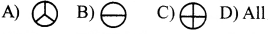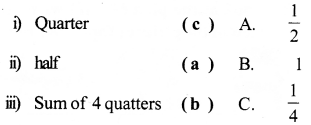# AP Board 3rd Class Maths Solutions 8th Lesson Share Equally

Andhra Pradesh AP Board 3rd Class Maths Solutions 8th Lesson Share Equally Textbook Exercise Questions and Answers.

## AP State Syllabus 3rd Class Maths Solutions Chapter 8 Share Equally

Textbook Page No. 96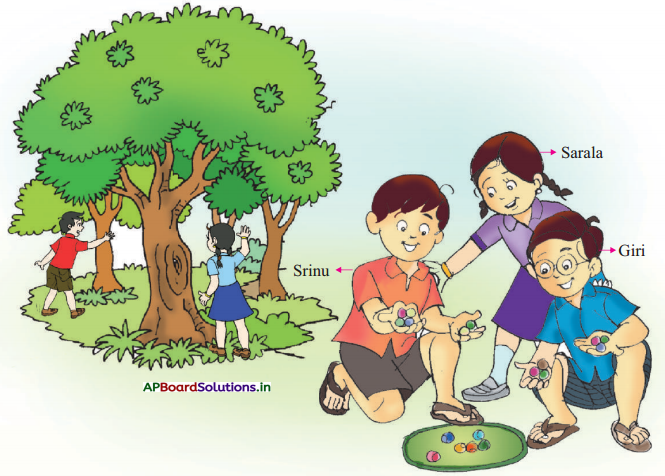I. Srinu and Giri are brothers. They are playing with their friends in a park. Observe the above picture and answer the following questions.

Question 1.
How many marbles does Srinu has?
6 marbles

Question 2.
How many marbles does Giri has?
6 marblesQuestion 3.
How many marbles are there in total?
6 + 6 = 12 marbles

Question 4.
Who has marble equally in both the hands ?
Srinu and Giri has

Question 5.
If the boys share all marbles equally how many marbles would each get ?
122 ÷ 2 = 6 marbles

Textbook Page No. 97

II. Srinu and Giri wanted to make a paper house. So, they brought 3 post cards and folded them in the middle as shown below.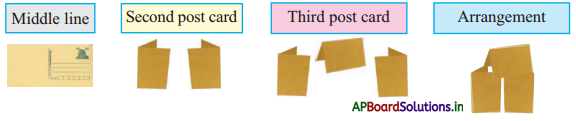Question 1.
How is the card folded into ? (Equal / not equal)
Equal

Question 2.
How the folded parts look like ? (Same/Not same)
Same

Question 3.
How many equal parts are each card folded into ?
two parts

Do these:

Observe the following pictures and put (‘✓ ‘) in the box, that have equal parts.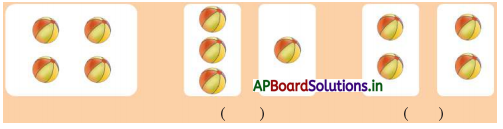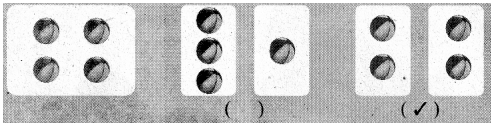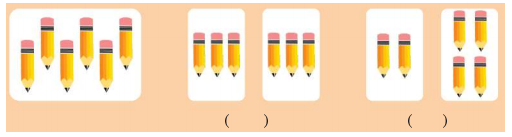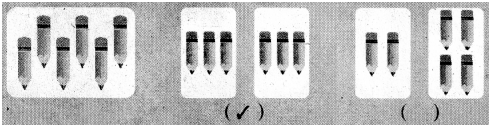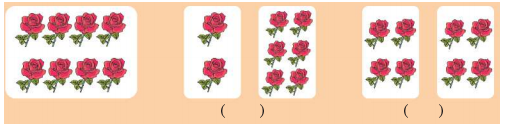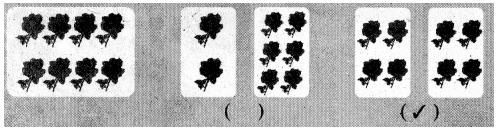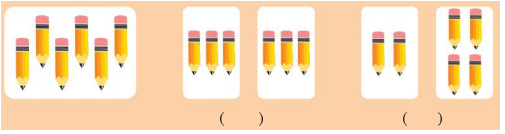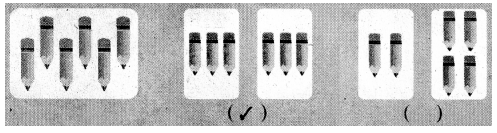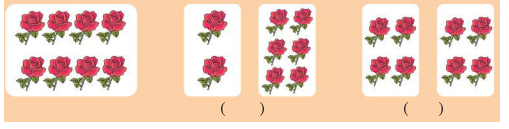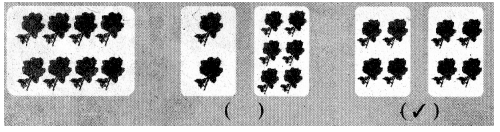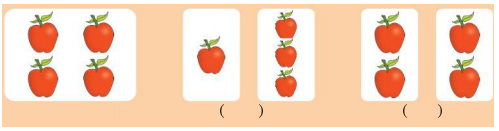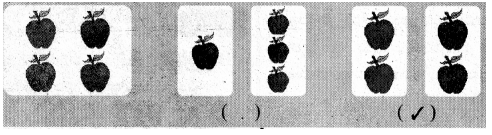Textbook Page No. 98

Colour half of the figure. One is done for you.
(Dotted line represents middle line)

a)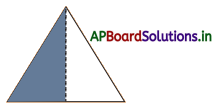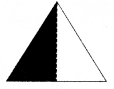b)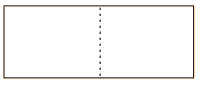c)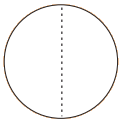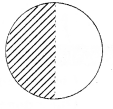d)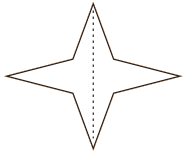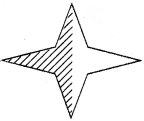Textbook Page No. 99

III. If Amma makes 3 dosas, how can she share them to Srinu and Girl equally?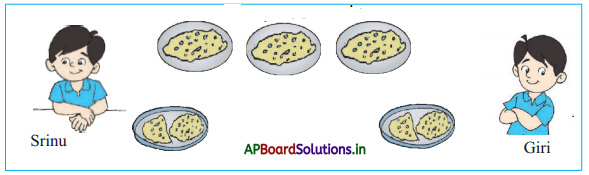Do Srinu and Girl get equal dosas?
Yes they got equally.

Do these
Share equally as expressed.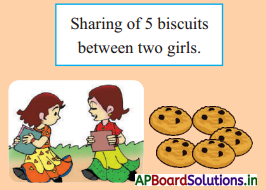2$$\frac{1}{2}$$ + 2$$\frac{1}{2}$$ part
2$$\frac{1}{2}$$ biscuits 2$$\frac{1}{2}$$ biscuits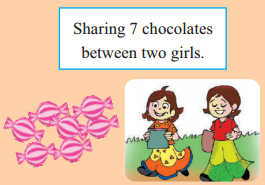3$$\frac{1}{2}$$ and 3$$\frac{1}{2}$$
3$$\frac{1}{2}$$ Chocolate 3$$\frac{1}{2}$$ Chocolate

Textbook Page No. 100

Do these:

I. Colour the quarter part of the figure.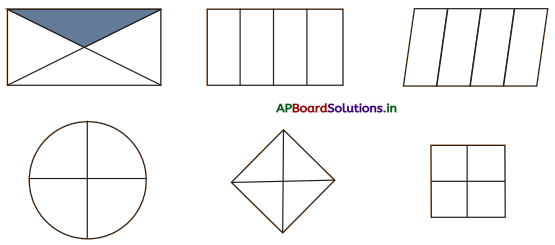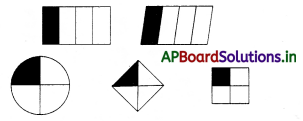II. Colour quarter number of objects in each picture.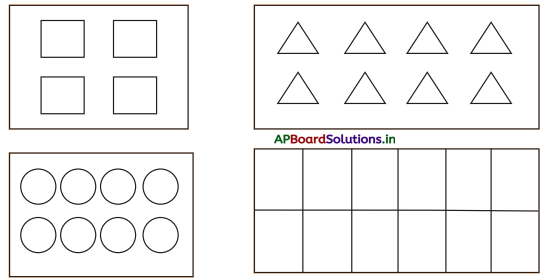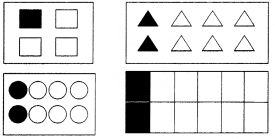Textbook Page No. 101

III. There are four members in a family. Share the land equally.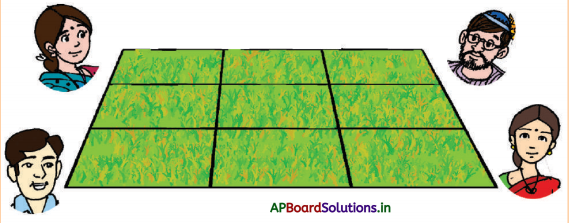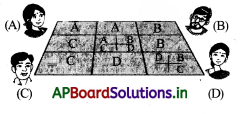IV. Share the buns equally among four monkeys.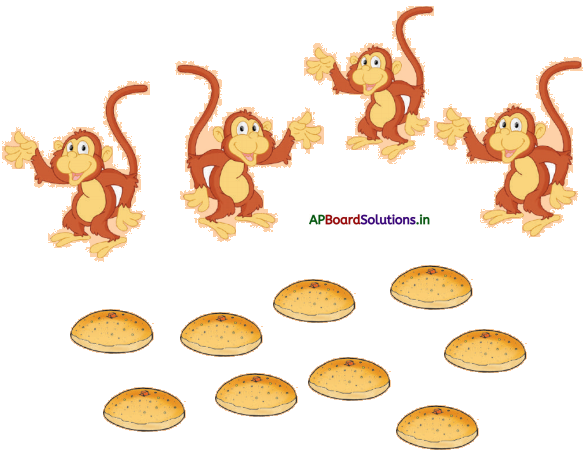Textbook Page No. 102

Exercise

1. Tick (✓) equally parted pictures.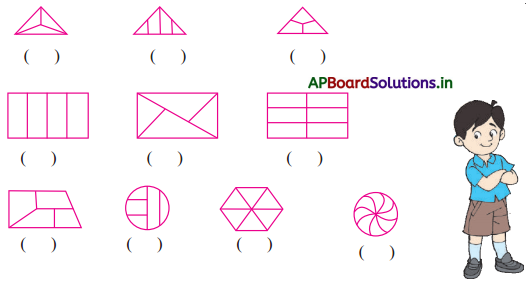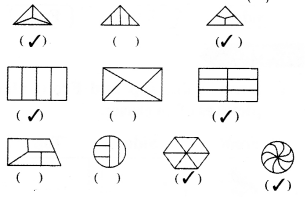II. Make 2 equal parts.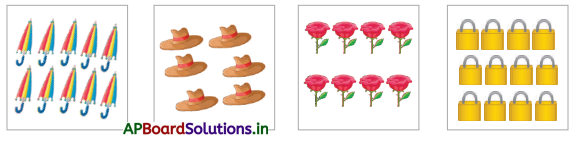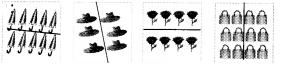III. Shade “half’ part of each picture.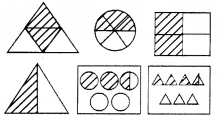IV. Shade “quarter” part of each picture.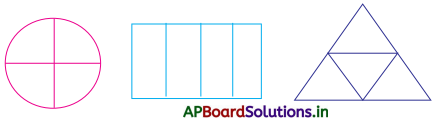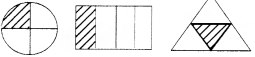Multiple Choice Questions

Question 1.
Half of half is called ( )
A) Full
B) quarter
C) Both A and B
D)None
B) quarter

Question 2.
Four quarter parts make one ( )
A) Full
B) quarter
C) Both A and B
D)None
A) FullQuestion 3.
Among the following equally shared ( )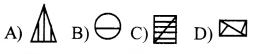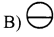Question 4.
Among the following quarterly shared ( )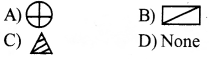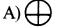Question 5.
If 32 biscuits are shared quarterly, then how many biscuits each on gets ( )
A) 16
B) 8
C) 24
D) 32
B) 8

Question 6.
Quarter is represented as ( )
A) 1
B) $$\frac{1}{4}$$
C) $$\frac{1}{2}$$
D) $$\frac{3}{4}$$
B) $$\frac{1}{4}$$